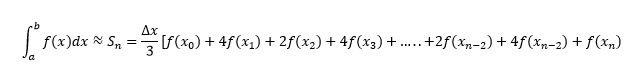# Simpson's Rule

Simpson’s rule is one of the numerical methods which is used to evaluate the definite integral. Usually, to find the definite integral, we use the fundamental theorem of calculus, where we have to apply the antiderivative techniques of integration. But sometimes it is difficult to find the antiderivative of an integral, like in the case of Scientific Experiments, where the function has to be determined from the observed readings. Therefore, the numerical methods are used to approximate the integral in such conditions. Other numerical methods used are trapezoidal rule, midpoint rule, left or right approximation using Riemann sums. Here, we are going to discuss Simpson’s rule formula, 1/3 rule, 3/8 rule, and examples.

## Simpson’s Rule Formula

Simpson’s rule methods are more accurate than the other numerical approximations and its formula for n+1 equally spaced subdivision is given by;Where n is the even number, △x = (b – a)/n and xi = a+i△x

If we have f(x) = y, which is equally spaced between [a,b] and if a = x0, x1 = x0 + h, x2 = x0 + 2h …., xn = x0 + nh, where h is the difference between the terms. Or we can say that y0 = f(x0), y1 = f(x1), y2 = f(x2),……,yn = f(xn) are the analogous values of y with each value of x.

## Simpson’s 1/3 Rule

Simpson’s 1/3rd rule is an extension of the trapezoidal rule in which the integrand is approximated by a second-order polynomial. Simpson rule can be derived from the various way using Newton’s divided difference polynomial,  Lagrange polynomial, and the method of coefficients. Simpson’s 1/3 rule is defined by:

 ∫ab f(x) dx = h/3[(y0+yn) + 4(y1+y3+y5+….+yn-1)+2(y2+y4+y6+…..+yn-2)]

This rule is known as Simpson’s One-third rule.

### Simpson’s ⅓ Rule for Integration

We can get a quick approximation for definite integrals when we divide a small interval[a,b] into two parts. Therefore, after dividing the interval, we get;

x0=a, x1= a+b, x2 = b

Hence, we can write the approximation as;

ab f(x) dx ≈ S2 = h/3[f(x0) + 4f(x1) + f(x2)]

S2 = h/3[f(a)+4f(a+b/2)+f(b)]

Where h = (b-a)/2

This is the simpson’s ⅓ rule for integration.

## Simpson’s 3/8 Rule

Another method of numerical integration method called “Simpson’s 3/8 rule”. It is completely based on the cubic interpolation rather than the quadratic interpolation. Simpson’s 3/8 or three-eight rule is given by:

 ∫ab f(x) dx = 3h/8[(y0+yn)+3(y1+y2+y4+y5+….+yn-1)+2(y3+y6+y9+…..+yn-3)]

This rule quite more accurate than the standard method, as it uses one more functional value. For 3/8 rule, the composite Simpson’s 3/8 rule also exists which is similar to the generalized form. The 3/8 rule is known as Simpson’s second rule of integration.

## Simpson’s Rule Error

Although in Simpson’s rule method we get a more accurate approximation for definite integral, still the error occurs which is defined as when n = 2;

-(1/90)(b-a/2)5f(4)(ξ)

Where ξ is some number between a and b.

## Simpson’s Rule Example

Example: Evaluate ∫01exdx, by Simpson’s ⅓ rule.

Solution:

Let us divide the range (0,1) into six equal parts by taking h = 1/6.

When, x0 = 0 then y0 = e0 = 1

Now, when;

x1 = x0 + h = ⅙, then y1 = e1/6 = 1.1813

x2 = x0 + 2h = 2/6 = 1/3 then, y2 = e1/3 = 1.3956

x3 = x0 + 3h = 3/6 = ½ then y3 = e1/2= 1.6487

x4 = x0 + 4h = 4/6 ⅔ then y4 = e2/3 = 1.9477

x5 = x0 + 5h = ⅚ then y5 = e5/6 = 2.3009

x6 = x0 + 6h = 6/6 = 1 then y6 = e= 2.7182

We know by Simpson’s ⅓ rule;

ab f(x) dx = h/3[(y0+yn) + 4(y1+y3+y5+….+yn-1)+2(y2+y4+y6+…..+yn-2)]

Therefore,

01exdx = 1/18[(1+2.718)+4(1.1813+1.6487+2.3009)+2(1.39561+1.9477)]

= 0.055[3.7182 + 20.52422 + 6.6866]

= 1.71828

Keep visiting BYJU’S – The Learning App for more Maths-related articles and download the app to learn the concepts with ease by watching interactive and engaging videos.